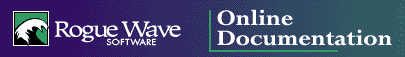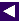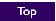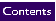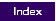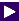## 5.2 vector Operations

In this section, each of the member functions provided by the vector datatype are described in more detail. These member functions provide the basic operations for vectors. They can be greatly extended through the generic algorithms described in Chapter 13.

### 5.2.1 Declaration and Initialization of vectors

Because it is a template class, the declaration of a vector must include a designation of the component type. This can be a primitive language type, like an integer or double, a pointer type, or a user-defined type. In the latter case, the user-defined type must implement a copy constructor, as this constructor is used to initialize newly created elements.

REMEMBER: Elements that are held by a vector must define a copy constructor. Although not used by functions in the vector class, some of the generic algorithms also require vector elements to recognize either the equivalence operator == or the relational less-than operator <.

Like an array, a vector is most commonly declared with an integer argument that describes the number of elements the vector will hold and an initial value for each element:

`vector<int> vec_one(10,0);`

For vectors as well as other Standard Library containers, an allocator type can also be provided as an additional template parameter:

`vector<int,allocator<int>>`

These two declarations are synonymous. Note that if the allocator template parameter is provided explicitly, then its type must match the contained type.

If the type has a default constructor, as it does in this case, then the second argument can be omitted. The constructor used to create the vector here is declared as explicit, which prevents it being used as a conversion operator. This is generally a good idea, since otherwise an integer might unintentionally be converted into a vector in certain situations.

There are a variety of other forms of constructor that can also be used to create vectors. If no size is provided, the vector initially contains no elements, and increases in size automatically as elements are added. The copy constructor creates a clone of a vector from another vector.

```vector<int> vec_two(5, 3);      // copy constructor
vector<int> vec_three;
vector<int> vec_four(vec_two);  //  initialization by assignment```

A vector can also be initialized using elements from another collection, by means of a beginning and ending iterator pair. The arguments can be any form of iterator; thus collections can be initialized with values drawn from any of the container classes in the Standard C++ Library that support iterators.

`vector <int> vec_five (aList.begin(), aList.end());`

NOTE: Because it requires the ability to define a method with a template argument different from the class template, some compilers may not yet support the initialization of containers using iterators. While compiler technology is catching up with the Standard C++ Library definition, the Rogue Wave version of the Standard C++ Library will support conventional pointers and vector iterators in this manner.

A vector can be assigned the values of another vector, in which case the target receives a copy of the argument vector.

`vec_three = vec_five;`

The assign() member function is similar to an assignment, but is more versatile and, in some cases, requires more arguments. Like an assignment, the existing values in the container are deleted, and replaced with the values specified by the arguments. There are two forms of assign(). The first takes two iterator arguments that specify a sub-sequence of an existing container. The values from this sub-sequence then become the new elements in the receiver. The second version of assign() takes a count and an optional value of the container element type. After the call the container holds only the number of elements specified by the count, which are equal to either the default value for the container type or the initial value specified.

```vec_six.assign(list_ten.begin(), list_ten.end());
vec_four.assign(3, 7);      // three copies of the value 7
vec_five.assign(12);        // twelve copies of value zero```

If a destructor is defined for the container element type, the destructor is called for each value removed from the collection.

Finally, two vectors can exchange their entire contents by means of the swap() operation. The argument container takes on the values of the receiver, while the receiver assumes those of the argument. A swap is very efficient, and should be used in preference to an explicit element-by-element transfer where appropriate.

`vec_three.swap(vec_four); `

### 5.2.2 Type Definitions

The class vector includes a number of type definitions, most commonly used in declaration statements. For example, an iterator for a vector of integers can be declared in the following fashion:

`vector<int>::iterator location;`

In addition to iterator, Table 8 defines the following types:

#### Table 8 -- Type definitions for class vector

Type Definition
value_type
The type associated with the elements the vector maintains.
const_iterator
An iterator that does not allow modification of the underlying sequence.
reverse_iterator
An iterator that moves in a backward direction.
const_reverse_iterator
A combination constant and reverse iterator.
reference
A reference to an underlying element.
const_reference
A reference to an underlying element that will not permit the element to be modified.
size_type
An unsigned integer type, used to refer to the size of containers.
difference_type
A signed integer type, used to describe distances between iterators.
allocator_type
The type of allocator used to manage memory for the vector.

### 5.2.3 Subscripting a vector

The value being maintained by a vector at a specific index can be accessed or modified using the subscript operator, just like an ordinary array. Also like arrays, there are no attempts to verify the validity of the index values. Indexing a constant vector yields a constant reference. Attempts to index a vector outside the range of legal values generates unpredictable and spurious results:

```cout << vec_five << endl;
vec_five = 17;```

The member function at() can be used in place of the subscript operator. It takes exactly the same arguments as the subscript operator, and returns exactly the same values, but it will throw an out-of-range exception if the argument is invalid.

The member function front() returns the first element in the vector, while the member function back() yields the last. Both also return constant references when applied to constant vectors.

`cout << vec_five.front() << " ... " << vec_five.back() << endl;`

### 5.2.4 Extent and Size-Changing Operations

In general, there are three different sizes associated with any vector.

• the number of elements currently being held by the vector

• the maximum size to which the vector can be expanded without requiring that new storage be allocated

• the upper limit on the size of any vector.

These three values are yielded by the member functions size(), capacity(), and max_size(), respectively:

```cout << "size: " << vec_five.size() << endl;
cout << "capacity: " << vec_five.capacity() << endl;
cout << "max_size: " << vec_five.max_size() << endl;```

The maximum size is usually limited only by the amount of available memory, or the largest value that can be described by the datatype size_type. The current size and capacity are more difficult to characterize. As noted in Section 5.2.5, elements can be added to or removed from a vector in a variety of ways. When elements are removed from a vector, the memory for the vector is generally not reallocated, and thus the size is decreased but the capacity remains the same. A subsequent insertion does not force a reallocation of new memory if the original capacity is not exceeded.

An insertion that causes the size to exceed the capacity generally results in a new block of memory being allocated to hold the vector elements. Values are then copied into this new memory using the assignment operator appropriate to the element type, and the old memory is deleted. Because this can be a potentially costly operation, the vector datatype provides a means for the programmer to specify a value for the capacity of a vector. The member function reserve() is a directive to the vector, indicating that the vector is expected to grow to at least the given size. If the argument used with reserve() is larger than the current capacity, a reallocation occurs and the argument value becomes the new capacity. (It may subsequently grow even larger; the value given as the argument need not be a bound, just a guess.) If the capacity is already in excess of the argument, no reallocation takes place. Invoking reserve() does not change the size of the vector, nor the element values themselves, with the exception that they may potentially be moved should reallocation take place.

`vec_five.reserve(20);`

A reallocation invalidates all references, pointers, and iterators referring to elements being held by a vector.

The member function empty() returns true if the vector currently has a size of zero, regardless of the capacity of the vector. Using this function is generally more efficient than comparing the result returned by size() to zero.

`cout << "empty is " << vec_five.empty() << endl;`

The member function resize() changes the size of the vector to the value specified by the argument. Values are either added to or erased from the end of the collection as necessary. An optional second argument can be used to provide the initial value for any new elements added to the collection, although this argument is not optional if the contained type does not have a default constructor. If a destructor is defined for the element type, the destructor is called for any values that are removed from the collection:

```
// become size 12, adding values of 17 if necessary
vec_five.resize (12, 17); ```

NOTE: A vector stores values in a single large block of memory. A deque, on the other hand, employs a numberof smaller blocks. This difference may be important on machines that limit the size of any single block of memory, because in such cases a deque will be able to hold much larger collections than a vector.

### 5.2.5 Inserting and Removing Elements

As noted earlier, the class vector differs from an ordinary array in that a vector can increase or decrease in size in certain circumstances. When an insertion causes the number of elements being held in a vector to exceed the capacity of the current block of memory being used to hold the values, a new block is allocated and the elements are copied to the new storage.

NOTE: Even adding a single element to a vector can, in the worst case, require time proportional to the number of elements in the vector, as each element is moved to a new location. If insertions are a prominent feature of your current problem, you should explore the possibility of using containers, such as lists or sets, which are optimized for insert operations.

A new element can be added to the back of a vector using the function push_back(). If there is space in the current allocation, this operation is very efficient (constant time).

`vec_five.push_back(21);   // add element 21 to end of collection`

The corresponding removal operation is pop_back(), which decreases the size of the vector, but does not change its capacity. If the container type defines a destructor, the destructor is called on the value being eliminated. Again, this operation is very efficient. The class deque permits values to be added and removed from both the back and the front of the collection, as described in Chapter 7.

More general insertion operations can be performed using the insert() member function. The location of the insertion is described by an iterator; insertion takes place immediately preceding the location denoted. A fixed number of constant elements can be inserted by a single function call. It is much more efficient to insert a block of elements in a single call than to perform a sequence of individual insertions, because with a single call at most one allocation will be performed.

```
// find the location of the 7
vector<int>::iterator where =
find(vec_five.begin(), vec_five.end(), 7);
// then insert the 12 before the 7
vec_five.insert(where, 12);
vec_five.insert(where, 6, 14);    // insert six copies of 14```

The most general form of the insert() member function takes a position and a pair of iterators that denote a sub-sequence from another container. The range of values described by the sequence is inserted into the vector. Again, using this function is preferable to using a sequence of individual insertions because at most a single allocation is performed.

`vec_five.insert (where, vec_three.begin(), vec_three.end());`

NOTE: Once more, it is important to remember that should an insertion cause reallocation, all references, pointers, and iterators that denoted a location in the now-deleted memory block become invalid.

In addition to the pop_back() member function, which removes elements from the end of a vector, a function exists that removes elements from the middle of a vector, using an iterator to denote the location. The member function that performs this task is erase(), which has two forms:

• the first takes a single iterator and removes an individual value

• the second takes a pair of iterators and removes all values in the given range. The size of the vector is reduced, but the capacity is unchanged. If the container type defines a destructor, the destructor is invoked on the eliminated values:

• ```
vec_five.erase(where);
// erase from the 12 to the end
where = find(vec_five.begin(), vec_five.end(), 12);
vec_five.erase(where, vec_five.end());```

### 5.2.6 Iteration

The member functions begin() and end() yield random access iterators for the container. Again, we note that the iterators yielded by these operations can become invalidated after insertions or removals of elements. The member functions rbegin() and rend() return similar iterators, but these access the underlying elements in reverse order. Constant iterators are returned if the original container is declared as constant, or if the target of the assignment or parameter is constant.

### 5.2.7 Test for Inclusion

A vector does not directly provide any method that can be used to determine if a specific value is contained in the collection. However, the generic algorithms find() or count() can be used for this purpose (see Section 13.3.1 and Section 13.6.1). For example, the following statement tests to see whether an integer vector contains the element 17:

`count (vec_five.begin(), vec_five.end(), 17);`

If your compiler does not support partial specialization, then you must use the following interface instead:

```int num = 0;
count (vec_five.begin(), vec_five.end(), 17, num);

if (num)
cout << "contains a 17" << endl;
else
cout << "does not contain a 17" << endl;```

NOTE: count() returns its result through an argument that is passed by reference. It is important that this value be properly initialized before invoking this function.

### 5.2.8 Sorting and Sorted vector Operations

A vector does not automatically maintain its values in sequence. However, a vector can be placed in order using the generic algorithm sort() (see Section 14.2). The simplest form of sort uses for its comparisons the less-than operator for the element type. An alternative version of the generic algorithm permits the programmer to specify the comparison operator explicitly. This can be used, for example, to place the elements in descending rather than ascending order:

```   // sort ascending
sort (aVec.begin(), aVec.end());

// sort descending, specifying the ordering function explicitly
sort (aVec.begin(), aVec.end(), greater<int>() );

// alternate way to sort descending
sort (aVec.rbegin(), aVec.rend());```

A number of the operations described in Section 14 can be applied to a vector holding an ordered collection. For example, two vectors can be merged using the generic algorithm merge() (see Section 14.6):

```// merge two vectors, printing output
merge (vecOne.begin(), vecOne.end(), vecTwo.begin(), vecTwo.end(),
ostream_iterator<int,char> (cout, " "));```

Sorting a vector also permits the more efficient binary search algorithms (see Section 14.5), instead of a linear traversal algorithm such as find().

### 5.2.9 Useful Generic Algorithms

Most of the algorithms described in Part IV can be used with vectors, but some are more useful than others. For example, the maximum value in a vector can be determined as follows:

```vector<int>::iterator where =
max_element (vec_five.begin(), vec_five.end());
cout << "maximum is " << *where << endl;```

Table 9 summarizes some of the algorithms that are especially useful with vectors:

#### Table 9 -- Generic algorithms useful with vectors

Algorithm Purpose
fill
Fill a vector with a given initial value
copy
Copy one sequence into another
generate
Copy values from a generator into a vector
find
Find an element that matches a condition
Find consecutive duplicate elements
search
Find a sub-sequence within a vector
max_element, min_element
Locate maximum or minimum element
reverse
Reverse order of elements
replace
Replace elements with new values
rotate
Rotate elements around a midpoint
partition
Partition elements into two groups
next_permutation
Generate permutations
inplace_merge
Inplace merge within a vector
random_shuffle
Randomly shuffle elements in vector
count
Count number of elements that satisfy condition
accumulate
Reduce vector to a single value
inner_product
Inner product of two vectors
equal
Test two vectors for pair-wise equality
lexicographical_compare
Lexical comparison
transform
Apply transformation to a vector
partial_sum
Partial sums of values